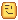# Math.tau as a builtin constant (2 * math.pi)

Minor request. It would be very helpful to have two pi as a constant value in the math library as it has many frequent uses in rotation and would remove a ton of (math.pi * 2) that need to be frequently written everywhere.

There’s lots of people that want this feature after many years of patiently waiting:18 Likes

Support, I’ve written `math.pi * 2` so many times I sometimes forget you’re supposed to multiply it by 2. Having `math.tau` would just simplify things and is certainly not a complex request

1 Like

I usually do the opposite, using math.pi / 2. I usually do this less, but I could see where it would find use.

This would be a great addition. Constants like this not only save time, but they also improve script performance (or so I’d assume).

in support of this but why not just make a plugin to which when you type `math.tau` it replaces it? until roblox adds it.

Just curious, what’s the use case for this? I almost never use math.pi, and I’ve never multiplied it by two specifically.

(Or divided, in this case)

pi is the ratio of the circumference of a circle to its diameter. Outside of engineering, diameter is very rarely used. tau is the ratio of the circumference to the radius. In most math and most especially in video games, radius is the more common measurement to work with. So tau is inherently more natural in the majority of cases.

1 Like

I recommend submitting an RFC Pull Request on the Luau repository as the math library is a part of luau and not just robloxhttps://github.com/Roblox/luau/pulls

1 Like

This will help a lot, hope Roblox adds it.

Just define it as a constant at the top of your code

``````math.tau = math.pi * 2
print(math.tau) -- > 6.2831853071796
``````

The Math library, and all other built-in libraries, are read-only tables and cannot be modified.

2 Likes

Here’s my question. Does referencing the math library take longer than just referencing a variable?
For example if you did a loop of

``````for x = 1,1000000 do
print(math.pi)
task.wait()  -- I know, this isn't an accurate timer, but you get my point
end
``````

would that take microseconds longer than

``````pi = 6.2831853071796
for x = 1,1000000 do
print(pi)
end
``````

If it takes longer then why not make a simple variable at the top of your script

``````tau = math.pi * 2
``````

as mentioned by @notjoshno, but without referencing the math library like @5P3C1A1_XD posted. This would also mean you don’t have to reference the math library each time you use it and typing `pi` or `tau` instead of `math.pi` or `math.tau` would be quicker.

How about `math.phi = (1 + math.sqrt(5)) / 2` while we’re at it? It’s used in many geometric formulae in particular, and takes longer to type out than tau by a fair bit.

1 Like

It would have in Lua 5.1, however Luau recognized that globals such as `math.pi` are extremely common, and Luau can index a global and up to two steps deeper in a single step, i.e. `game.Workspace.Part` takes only one step in Luau. This is for globals specifically. I don’t believe this works with any variables you create. The specific opcode is named `getglobal`.

Regardless of performance, the ‘why not’ from our perspective is ‘why not add it’ since it can conceivably be implemented bug-free in a matter of minutes, so long as everyone is on board with the changes.

3 Likes

Ah, verified this in the lua demo tool (Lua: demo) as I was on mobile.

In which case, simply assign it to a variable name?

``````local tau = math.pi*2
``````

I think the math library is low level and uses C functions to have massive performance boosts

That’s true. The compiler for the math luau library (not lua) can be found here: luau/lmathlib.cpp at master · Roblox/luau · GitHub.

Enjoy.``````// ADD
#undef TAU
#define TAU (6.28318530718)
``````
1 Like

I already made a pull request for that1 Like

Appreciate you making a pull request for the math.tau feature request <3

1 Like

Do you know any use cases for the `math.tau` thing?

Its in the topic itself.